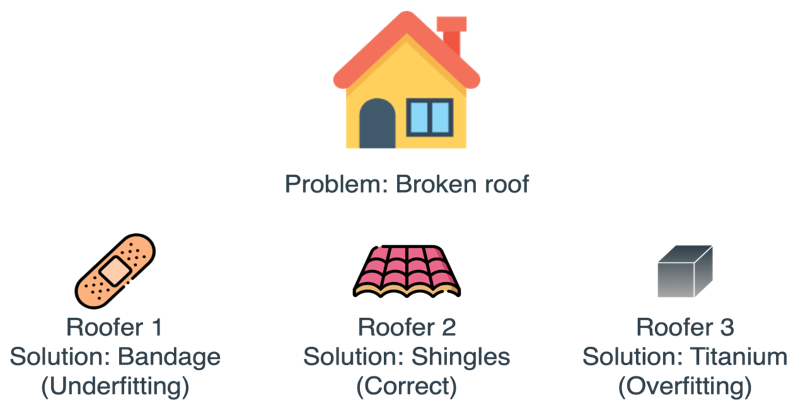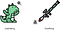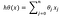# Regularization in Machine Learning— Explained!Image Credits: https://livebook.manning.com/

I must admit that this particular concept haunted me almost every time I had a model that worked great on the training data, but failed miserably on unseen data. Although most data professionals use this technique to better generalize their models, but the intuition behind the application of this technique is often lost behind the algorithms and codes.

So I thought of writing about this amazing technique and the arsenal of firearms in its possession.

# Definition:

Regularization is a technique which is used to solve the overfitting problem of machine learning models.

Now comes the inevitable question!
What is Overfitting?
Overfitting is a phenomenon which occurs when a model learns the detail and noise in the training data to an extent that it negatively impacts the performance of the model on new data. So, overfitting is a major problem as it negatively impacts the performance of the model.
We need to build our models such that they generalize well on the new data. Generalization refers to our model’s ability to adapt properly to new, previously unseen data, drawn from the same distribution as the one used to create the model.Image Credits: https://livebook.manning.com/

Regularization helps us to maintain all variables or features in the model by reducing the magnitude of the variables. Hence, it maintains accuracy as well as the generalization power of the model.

Let us now dive into some simple mathematics to understand how regularization helps to solve the overfitting problem. The lambda parameter controls the amount of regularization applied to the model.

Say, we have a set of points (x1,y1),(x2,y2),(x3,y3),…..,(xn,yn). Our goal is to fit a function to the given points such that y = f(x). The hypothesis for this problem is:where x is a vector [x1,x2,x3,x4,x5,..….,xn]

We are trying to find the values of θ’s such that the function outputs a value close to given y values. Using least square error to compute our cost function, we have

A machine learning model is said to be complex when the model has so many Thetas, that model memorizes everything in the data — signals and the noise. Such a model will perform well on the training data, but will fail to generalize on the test or unseen data. This is the issue of overfitting.
The cost function should be zero if our hypothesis has an output same as y. But the model might overfit the training data, and might not generalize well for the test data . So we want to penalize all theta values to reduce the model complexity.

Now let’s dig deep more into our cost function:

## Without Regularization:

It can be written in vectorized form as

Expanding it

Considering it as a quadratic equation in θ , it will tend to zero at its roots.

## With Regularization:

Adding regularization to our cost function, it becomes

Solving for roots

We see that only constant a has changed(increased) in the regularization equation, thereby causing a decrease in D and an increase in N . Hence, the overall value of theta decreases.

# Types of regularizations in Machine Learning

Two of the commonly used techniques are L1 or Lasso regularization and L2 or Ridge regularization. Both these techniques impose a penalty on the model to achieve dampening of the magnitude or amplitude of the features. In the case of L1, the sum of the absolute values of the weights is imposed as a penalty while in the case of L2, the sum of the squared values of weights is imposed as a penalty. There is a hybrid type of regularization called Elastic Net that is a combination of L1 and L2.

## When to use which Regularization technique?

The next issue is to decide on the type of regularization technique one is going to need in a model. The two types of regularization technique work in slightly different ways. L1 is usually preferred when we are interested in fitting a linear model with fewer variables. L1 seems to encourage the coefficients of the variables to go towards zero because of the shape of the constraint which is an absolute value.

L1 is also useful when considering a categorical variable with many levels. L1 would make many of variable/feature weights go towards zero and thus leaving only the important ones in the model. This also helps in feature selection. L2 does not encourage convergence towards zero but is likely to make them closer to zero and prevent overfitting. Ridge or L2 is useful when there are a large number of variables with relatively smaller data samples, like in the case of genomic data.

These techniques will be explained in some other article, where we will see their explanation and implementation in Python.

Thanks for reading! Stay Safe!

Is a pantomath and a former entrepreneur. Currently, he is in a harmonious and a symbiotic relationship with Data.

## More from Aman Gupta

Is a pantomath and a former entrepreneur. Currently, he is in a harmonious and a symbiotic relationship with Data.